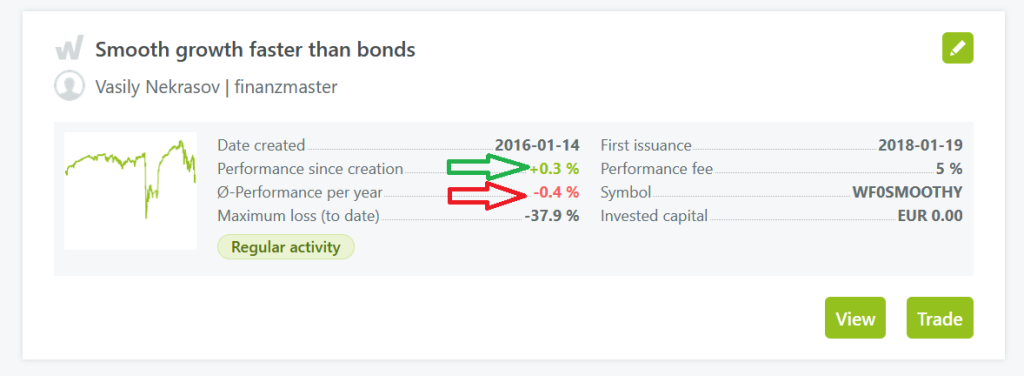## Wikifolio – Yet another fuckup – They cannot even a school math

Just had a look at one of my Wikifolios: the overall performance is positive but the annualized one is negative. Sorry guys (and gals): it is impossible, since the total performance is calculated according to$(1+r)^t$, where$r$ is the annual[ized] return, an$t$ is the [fractional] number of years (and the latter is obviously positive). Even if you use the formula of continuous rate$\exp{(rt)}$ (which you definitely do not understand since it is slightly beyond the school math), it still cannot be so that$r$ is negative but the overall performance is positive.Continue reading "Wikifolio – Yet another fuckup – They cannot even a school math"